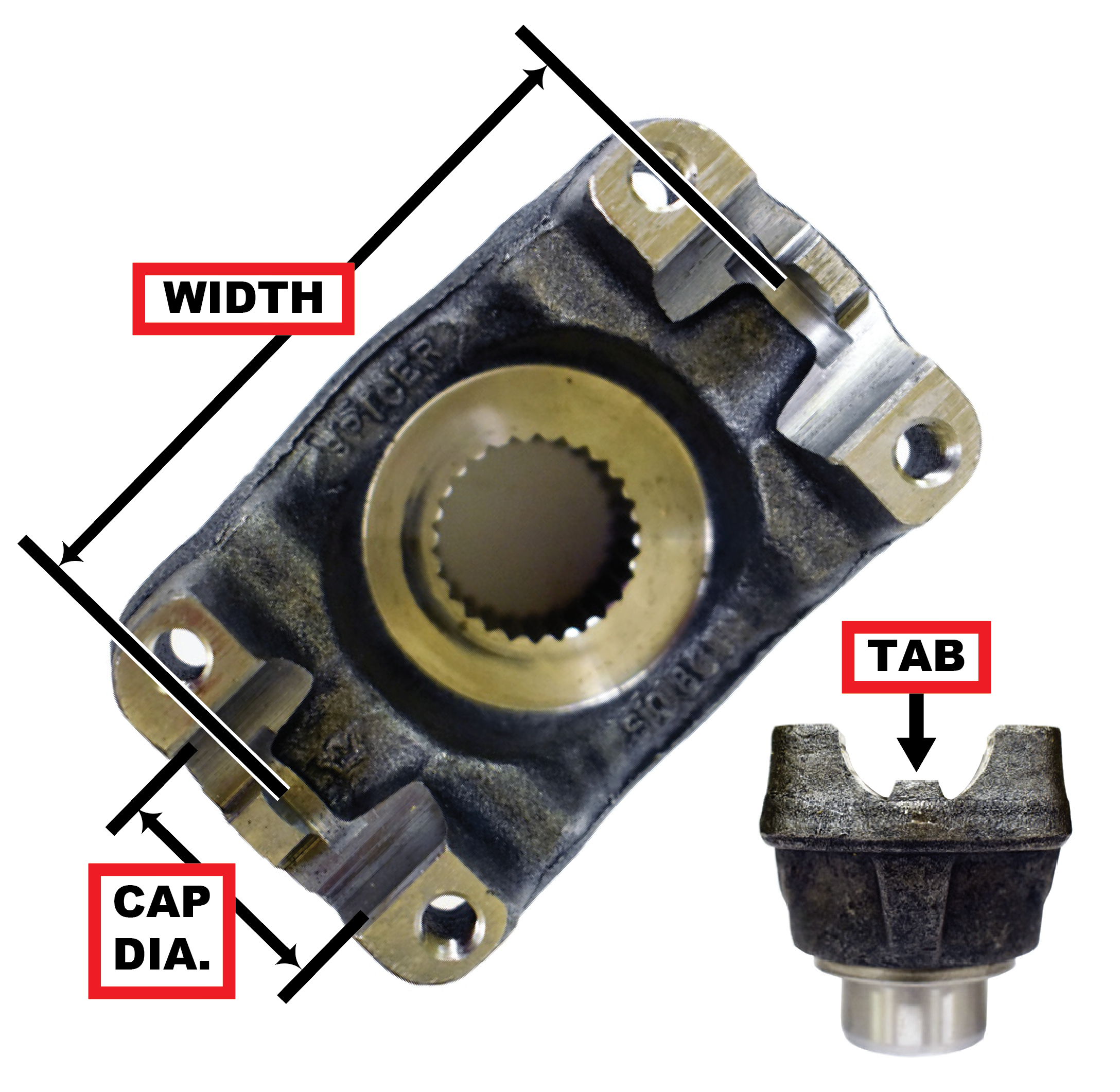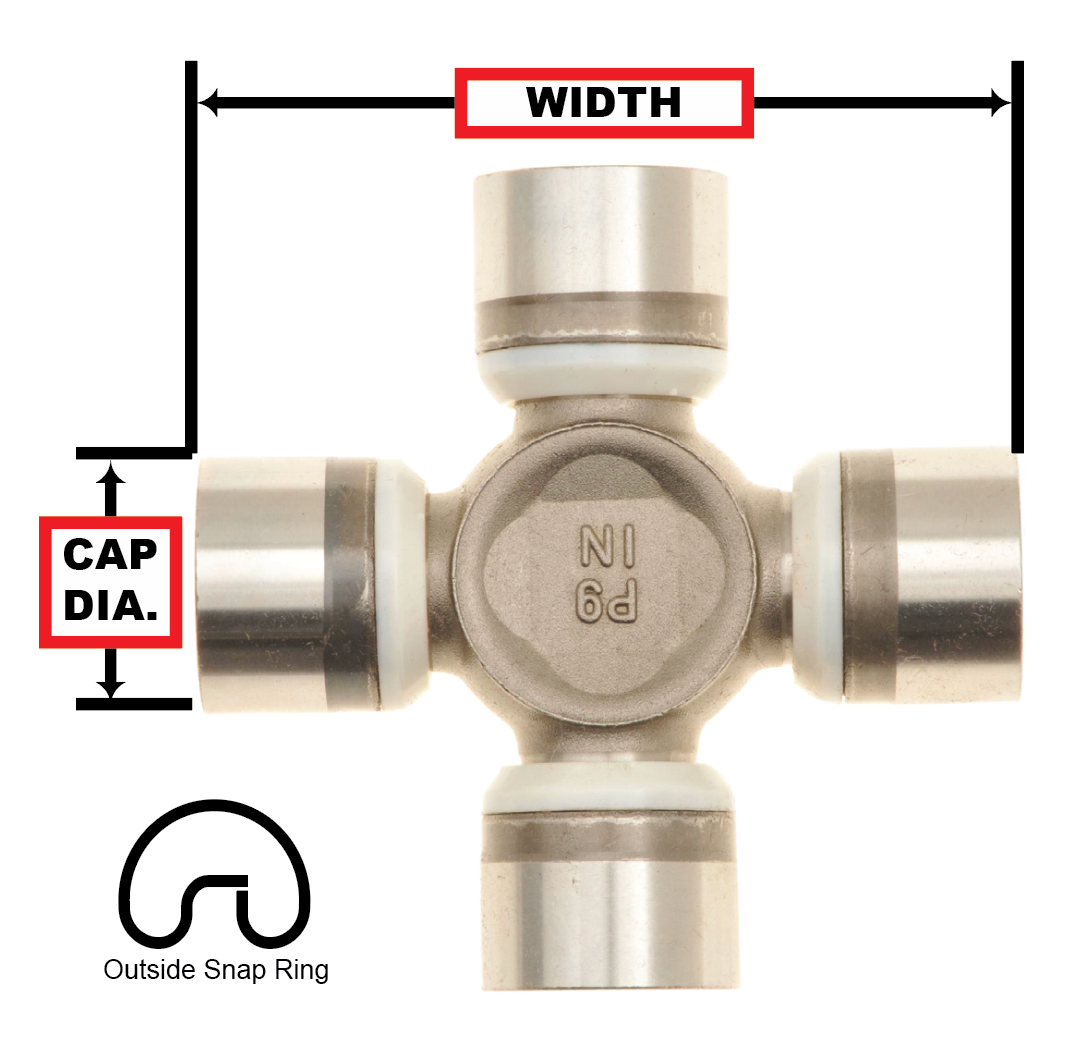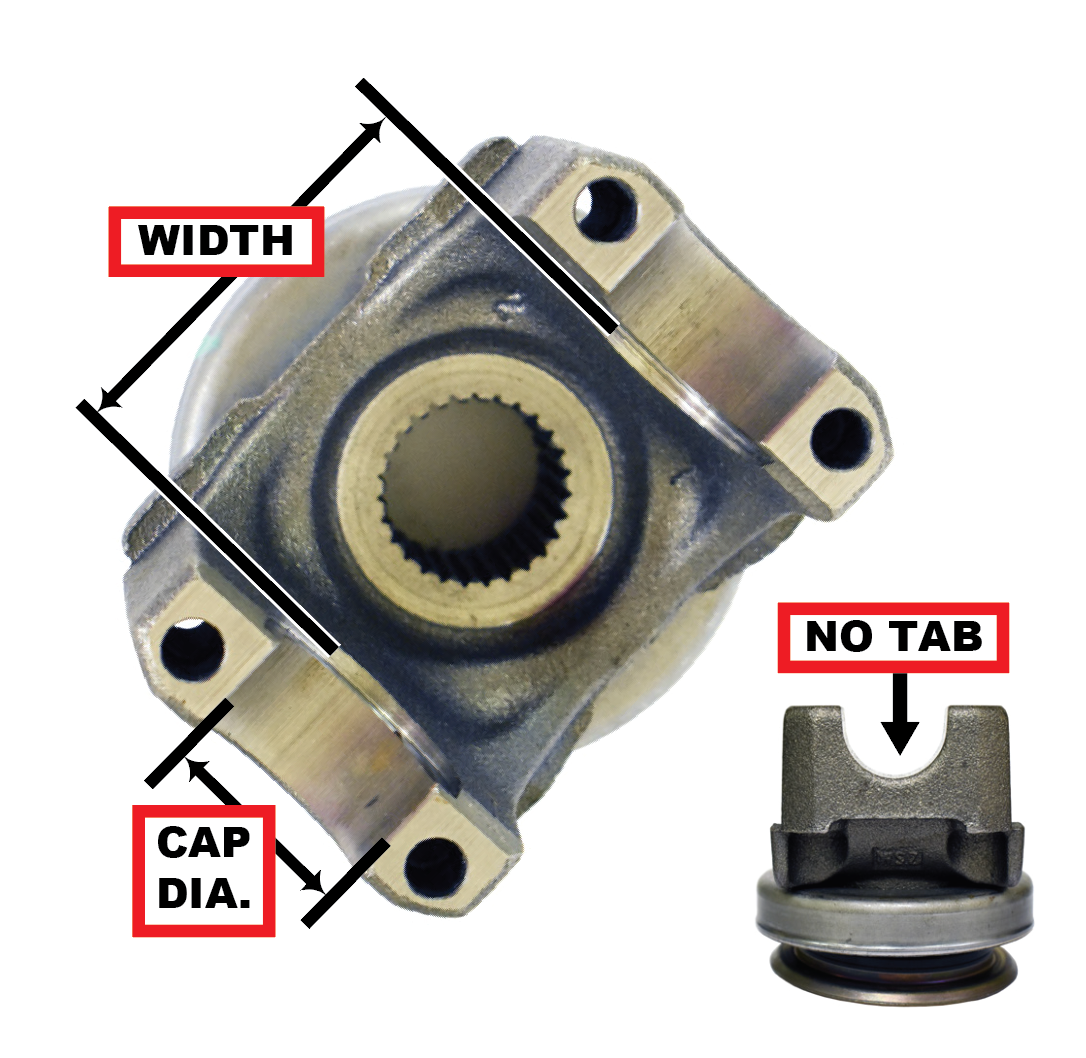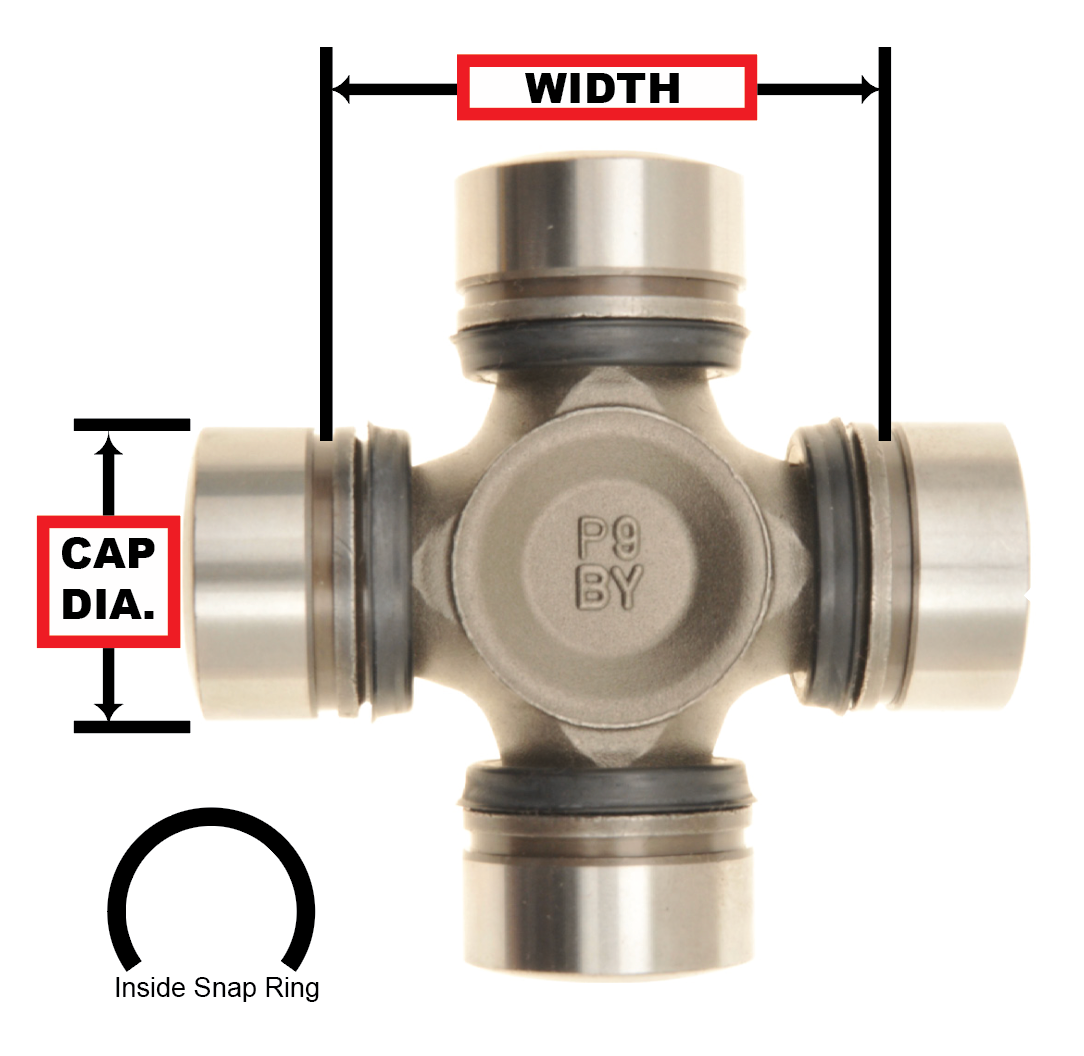# End Yoke Identifier

### Use this guide if you don't know your pinion, transfer case model or u-joint size.A.) Spline Count B.) Spline Outside Diameter (from farthest point to farthest point) C.) Hub Outside Diameter D.) Overall Height E.) Through Hole

### Are you looking for an inside snap ring or outside snap ring style end yoke?# Outside Snap Ring Style End YokeF.) Width: Distance measured between end yoke tabs. G.) Cap Diameter:Diameter of bearing cap.

### Common Outside Snap Ring U-joint Sizes and Series

Series Width Cap Diameter.
1210 2 7/16" = 2.438 1 1/16" = 1.063
1310 3 7/32" = 3.219 1 1/16" = 1.063
1310 Ford*
3 7/32" = 3.219
1 1/8" = 1.125
1330 3 5/8" = 3.625 1 1/16" = 1.063
1330 Ford* 3 5/8" = 3.625
1 1/8" = 1.125
1350 3 3/8" = 3.625 1 3/16" = 1.188
1410 4 3/16 = 4.188
1 3/16" = 1.188
1415** 4.178 1 3/16" = 1.188
1480 4 3/16 = 4.188 1 3/8" = 1.375
1485** 4.178 1 3/8" = 1.375
1550 4 31/32" = 4.969 1 3/8" = 1.375
1555** 4.964 1 3/8" = 1.375
* 1310 Ford & 1330 Ford have 1 1/8” cap at pinion yoke and 1 1/16” cap at driveshaft.
** 1415, 1485, 1555 only found on Chrysler and some GM vehicles with American Axle built driveshafts.

# Inside Snap Ring Style End YokeF.) Width: Distance measured machined flats. G.) Cap Diameter:Diameter of bearing cap.

### Common Inside Snap Ring U-joint Sizes and Series

Series Width Cap Diameter.
7260 Chrysler 2 1/8" = 2.125 1 5/64" = 1.078
7290 Chrysler 2 5/8" = 2.625 1 1/8" = 1.125
3R GM  2 9/16" = 2.563 1 1/8" = 1.125